#Nonlinear Second Order Differential EquationsIn general, little is known about nonlinear second order differential equations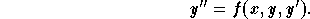,

but two cases are worthy of discussion:

(1)
Equations with the y missing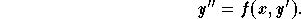Let v = y'. Then the new equation satisfied by v is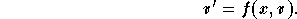This is a first order differential equation. Once v is found its integration gives the function y.

Example 1: Find the solution of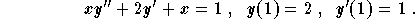Solution: Since y is missing, set v=y'. Then, we have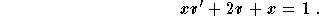This is a first order linear differential equation. Its resolution gives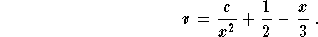Since v(1) = 1, we get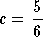. Consequently, we have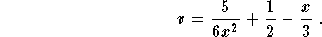Since y'=v, we obtain the following equation after integration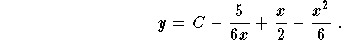The condition y(1) = 2 gives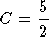. Therefore, we have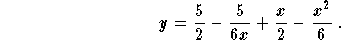Note that this solution is defined for x > 0.

(2)
Equations with the x missing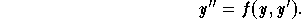Let v = y'. Since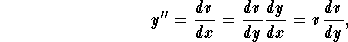we get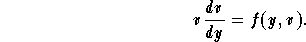This is again a first order differential equation. Once v is found then we can get y through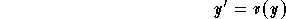which is a separable equation. Beware of the constants solutions.

Example 2: Find the general solution of the equation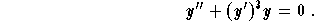Solution: Since the variable x is missing, set v=y'. The formulas above lead to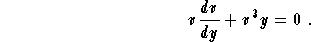This a first order separable differential equation. Its resolution gives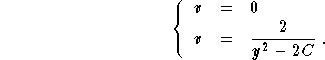Since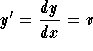, we get y' = 0 or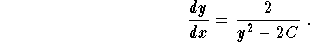Since this is a separable first order differential equation, we get, after resolution,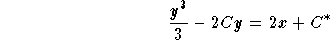,

where C and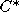are two constants. All the solutions of our initial equation are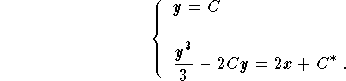Note that we should pay special attention to the constant solutions when solving any separable equation. This may be source of mistakes...[Differential Equations] [First Order D.E.] [Second Order D.E.]
[Geometry] [Algebra] [Trigonometry ]
[Calculus] [Complex Variables] [Matrix Algebra]S.O.S MATHematics home page

Do you need more help? Please post your question on our S.O.S. Mathematics CyberBoard.Author: Mohamed Amine Khamsi